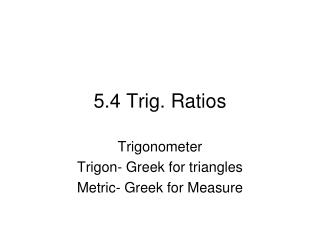DownloadDownload Presentation5.4 Trig. Ratios

# 5.4 Trig. Ratios

Download Presentation## 5.4 Trig. Ratios

- - - - - - - - - - - - - - - - - - - - - - - - - - - E N D - - - - - - - - - - - - - - - - - - - - - - - - - - -
##### Presentation Transcript

1. 5.4 Trig. Ratios Trigonometer Trigon- Greek for triangles Metric- Greek for Measure

2. Trig Ratios Trig. Ratios are used to find…… • A missing side on a right triangle using one given side and an angle. Ex: 3cm

3. Trig Ratios Continued … Trig Ratios are also used to find… • A missing angle on a right triangle using two given two sides. Ex. 3cm 4cm

4. Identifying sides of a triangle • Sides of a triangle are referenced to the angle Adjacent Hypotenuse Opposite

5. What if the angle is in the other corner? • Sides are identified according to the reference angle. Opposite Hypotenuse Adjacent

6. Trig Ratios Continued… • Trig Ratios are constant values of right triangles that are based on the ratios of side measurements.

7. SOH CAH TOA

8. 5cm 13cm 12cm If you want to find the angle in degrees you can use your calculator or a chart Trig Ratios

9. We already found this information Make sure your calculator is in degrees. Punch in 2nd Function sin(.3846) Your answer should be 22.62 degrees Punch in 2nd Function cos(.9231) and your answer should still be 22.62 degrees And the same thing if you do it for Tan Finding the Angle in Degrees

10. 8cm 12cm What are we going to use Sin, Cos or Tan? We have to use Sin WHY? We have to use sin because we have the opposite side and the hypotenuse Finding the angle given 2 sides of a triangle

11. 6 15 What ratio are we going to use sin cos or tan? We have to use cos because we have the adjacent side and the hypotenuse side. Finding the angles of a triangle

12. Finding two angles given 2 sides 15 30 What do we use sin, cos or tan when looking for the angles?

13. 9 15 8 20 Find the missing Angle

14. Solutions Example #1 Example #2

15. Trig Ratios Part 2 You have to decide what formula you are going to use Sin, Cos or Tan Lets use Now solve for y by multiplying both sides by 15 • Using Ratios to find the missing side. y X 15

16. Trig Ratios Part 2 You have to decide what formula you are going to use to solve for x Sin, Cos or Tan Lets use cos We have to move the x to the other side if we want to solve for X so to do this we must multiply both sides by x. The x’s will cancel on the right and now we must divide both sides by cos(45) • Using Ratios to find the missing side. y X 15

17. Solving for Sides VS Angles • When solving for a side you have to use the function cos, sin, and tan • When solving for an angle you need to use the inverse function (On your calculator you must push 2nd function first)

18. Solving for a Side • Find the missing side 12 x 30 y • What are we going to use to find x SOH CAH TOA

19. Solving for a Side • Find the missing side 12 x 30 y • What are we going to use to find y SOH CAH TOA